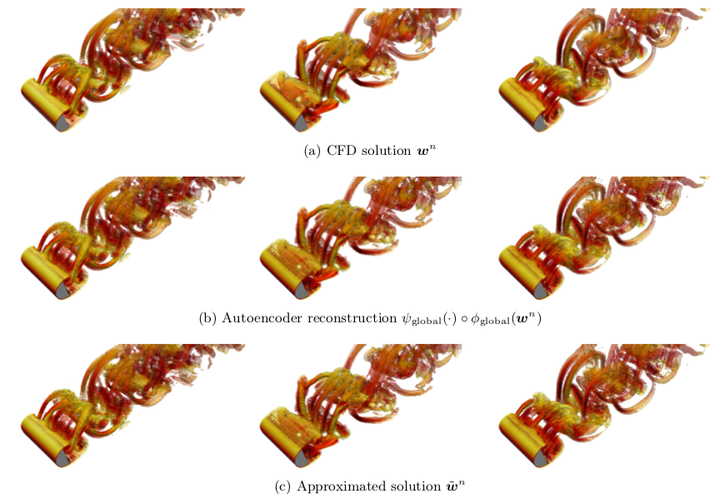# Recovering missing CFD data for high-order discretizations using deep neural networks and dynamics learningInstantaneous iso-surfaces of Q-criterion colored by velocity magnitude at $n =1000$, $4 000$, and $7 000$.

### Abstract

Data I/O poses a significant bottleneck in large-scale CFD simulations; thus, practitioners would like to significantly reduce the number of times the solution is saved to disk, yet retain the ability to recover any field quantity (at any time instance) a posteriori. The objective of this work is therefore to accurately recover missing CFD data a posteriori at any time instance, given that the solution has been written to disk at only a relatively small number of time instances. We consider in particular high-order discretizations (e.g., discontinuous Galerkin), as such techniques are becoming increasingly popular for the simulation of highly separated flows. To satisfy this objective, this work proposes a methodology consisting of two stages: 1) dimensionality reduction and 2) dynamics learning. For dimensionality reduction, we propose a novel hierarchical approach. First, the method reduces the number of degrees of freedom within each element of the high-order discretization by applying autoencoders from deep learning. Second, the methodology applies principal component analysis to compress the global vector of encodings. This leads to a low-dimensional state, which associates with a nonlinear embedding of the original CFD data. For dynamics learning, we propose to apply regression techniques (e.g., kernel methods) to learn the discrete-time velocity characterizing the time evolution of this low-dimensional state. A numerical example on a large-scale CFD example characterized by nearly 13 million degrees of freedom illustrates the suitability of the proposed method in an industrial setting.

Publication
Journal of Computational Physics, Vol. 395, p. 105–124 (2019)